# How to Add / Subtract Days, Months and Years to Date

This post will guide you how to add or subtract a given number of days to a date in excel. How to add or subtract weeks to a date using excel formula. How to add or subtract a certain number of months to a date using excel formula. How to add or subtracts a given number of years to a date using an excel formula.  And how to add or subtract a combination of years, months and days to a date.

Assuming that you have a list of date in your worksheets, and you want to perform some arithmetic operations (such as, addition or subtraction) to calculate different time units, such as, you want to add or subtract a number of days to or from a date by using a formula to adjust the date according to your needs.

## Add / subtract days to date

If you have a list of date in a range or worksheet, and you want to add or subtract a certain number of days to the date in the range, you can refer to the following general formula:

`=Date + days`

Or

`=Date - days`

For example, to add 100 days to a date in Cell B1, then you can write the following formula in Cell C1:

=B1 +100

To subtract 100 days from a date in Cell B1, then you can write the following formula in Cell D1

=B1-100

If you want to add or subtract 100 days to a date in Cell b1, then you can use the TODAY function to build the following formula:

=Today() +100

Or

=Today() – 100

Let’s see the below screenshot to see the returned result by the above formulas: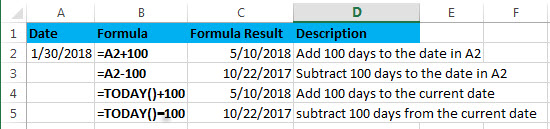## Add / subtract weeks to date

Assuming that you want to add or subtract a given number of weeks to a certain date in A2, then you can refer to the following generic formula:

`=Date +N Weeks * 7  `

Or

`=Date – N weeks*7`

You need to multiply the number of weeks by 7 to get the total number of days, then you can perform arithmetic operations in the same time unit (days).

Let’s see the below examples:

Supposing that you want to add or subtract 5 weeks to a date in A2, you can refer to the following excel formulas:

=A2 + 5*7

=A2 – 5*7

=TODAY() + 5*7

=TODAY() – 5*7

Let’s see the returned results by the above formula from the below screenshot: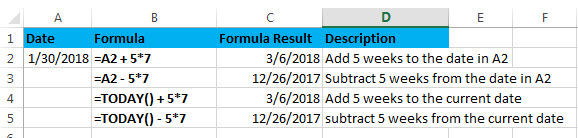## Add / subtract months to date

If you want to add or subtract a given number of whole months to a date, you can use the EDATE function to create a generic formula as follows:

`=EDATE(start_date, months)`

Start_date – the start date

Months – the number of months that you want to add or subtract

If you want to subtract months, you just need to enter a negative number as the months argument in the EDATE function.

Assuming that you want to add or subtract 3 months to the date in A2, you can use the following formula:

=EDATE(A2,3)

=EDATE(A2,-3)

=EDATE(TODAY(),3)

=EDATE(TODAY(),-3)

Let’s see the last result for above formulas: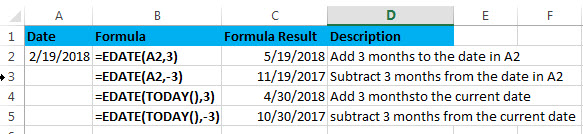## Add / subtract years to date

If you want to add or subtract a given number of years to a date, you can create an excel formula based on the DATE function, the YEAR function, the MONTH function and the DAY function.

The generic formula is as follows:

To add years to a date in excel:

`=DATE(YEAR(date)+ years, MONTH(date),DAY(date))`

To subtract years to a date in excel:

`=DATE(YEAR(date)+ years, MONTH(date),DAY(date))`

Assuming that you want to add or subtract 2 years to a date in A2, you can use the following formulas:

=DATE(YEAR(A2)+ 2, MONTH(A2),DAY(A2))

or

=DATE(YEAR(A2)- 2, MONTH(A2),DAY(A2))

Let’s see how this formula works:

=YEAR(A2)

Result: 2018

The YEAR function returns the year component of a date in A2.

=MONTH(A2)

Result: 2

The MONTH function returns the month portion of a date in A2.

=DAY(A2)

Result: 19

The DAY function returns the day of component of a date in A2.

Let’s see the following screenshot to show the result for above DATE formulas: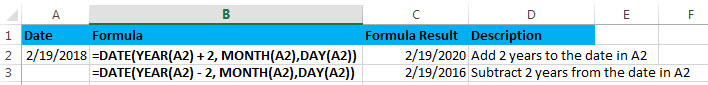## Add / subtract a combination of days, months and years

If you want to add or subtract a combination of days, months and years to a date in a single formula, you can also use a combination of the DATE function, the YEAR function, the MONTH function and the DAY function.

To add days, months and years in excel:

`=DATE(YEAR(start_date) + years, MONTH(start_date) + months, DAY(start_date) + days)`

To subtract days, months and years

`=DATE(YEAR(start_date) - years, MONTH(start_date) - months, DAY(start_date) - days)`

Assuming that you want to add or subtract 2 years, 3 months and 100 days to or from a date in cell A2, you can use the following formulas:

=DATE(YEAR(A2) + 2, MONTH(A2) + 3, DAY(A2) + 100)

Or

=DATE(YEAR(A2) – 2, MONTH(A2) – 3, DAY(A2) – 100)

Then enter the above formula into Cell C2 or C3, then let’s see the returned results: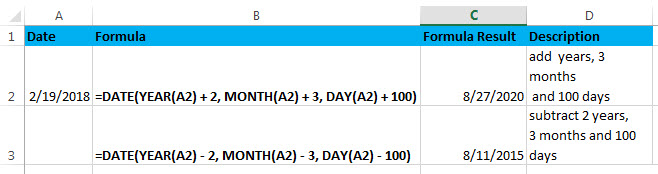### Related Formulas

• Convert date to month and year only in excel
If you want to convert the date to month and year only, you can use “yyyymm” format code within the TEXT function in excel, so you can write down the below TEXT formula:=TEXT(date,”yyyymm”)
• Convert date to month and day only in excel
If you want to convert the date (mm/dd/yyyy) to month and day only, you can use “mm dd” format code within the TEXT function in excel, so you can write down the below TEXT formula: =TEXT(date,”mm dd”)
• Convet Text Date dd/mm/yy to mm/dd/yyyy as Date Format
If you want to convert dates in the text format to the date format as mm/dd/yyyy, you can use a combination of the DATE function, the VALUE function, the RIGHT function, the MID function, and the LEFT function to create a new excel formula…

### Related Functions

• Excel DAY function
The Excel DAY function returns a day of a date (from 1 to 31).The DAY function is a build-in function in Microsoft Excel and it is categorized as a DATE and TIME Function.The syntax of the DAY function is as below:= DAY (date_value)…
• Excel EDATE function
TThe Excel EDATE function returns the serial number that represents the date that is a specified number of months before or after a specified date.The syntax of the EDATE function is as below:=EDATE (start_date, months)…
• Excel DATE function
The Excel DATE function returns the serial number for a date.The syntax of the DATE function is as below:= DATE (year, month, day)…
• Excel TODAY function
The Excel TODAY function returns the serial number of the current date. So you can get the current system date from the TODAY function. The syntax of the TODAY function is as below:=TODAY()…
• Excel MONTH Function
The Excel MONTH function returns the month of a date represented by a serial number. And the month is an integer number from 1 to 12.The syntax of the MONTH function is as below:=MONTH (serial_number)…